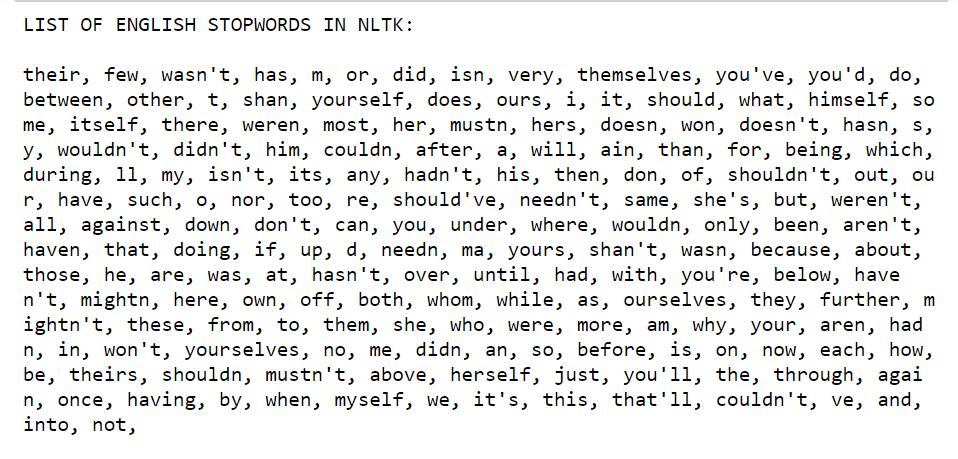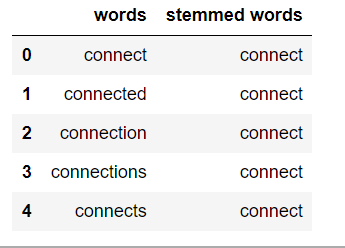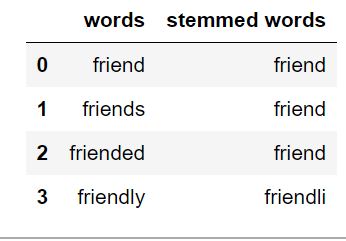Related Articles

# Text Preprocessing in Python | Set – 1

• Difficulty Level : Basic
• Last Updated : 26 Apr, 2020

Prerequisites: Introduction to NLP

Whenever we have textual data, we need to apply several pre-processing steps to the data to transform words into numerical features that work with machine learning algorithms. The pre-processing steps for a problem depend mainly on the domain and the problem itself, hence, we don’t need to apply all steps to every problem.

In this article, we are going to see text preprocessing in Python. We will be using the NLTK (Natural Language Toolkit) library here.

 `# import the necessary libraries``import` `nltk``import` `string``import` `re`

### Text Lowercase:

We lowercase the text to reduce the size of the vocabulary of our text data.

 `def` `text_lowercase(text):``    ``return` `text.lower()`` ` `input_str ``=` `"Hey, did you know that the summer break is coming? Amazing right !! It's only 5 more days !!"``text_lowercase(input_str)`

Example:

Input: “Hey, did you know that the summer break is coming? Amazing right!! It’s only 5 more days!!”
Output: “hey, did you know that the summer break is coming? amazing right!! it’s only 5 more days!!”

### Remove numbers:

We can either remove numbers or convert the numbers into their textual representations.
We can use regular expressions to remove the numbers.

 `# Remove numbers``def` `remove_numbers(text):``    ``result ``=` `re.sub(r``'\d+'``, '', text)``    ``return` `result`` ` `input_str ``=` `"There are 3 balls in this bag, and 12 in the other one."``remove_numbers(input_str)`

Example:

Input: “There are 3 balls in this bag, and 12 in the other one.”
Output: ‘There are balls in this bag, and in the other one.’

We can also convert the numbers into words. This can be done by using the inflect library.

 `# import the inflect library``import` `inflect``p ``=` `inflect.engine()`` ` `# convert number into words``def` `convert_number(text):``    ``# split string into list of words``    ``temp_str ``=` `text.split()``    ``# initialise empty list``    ``new_string ``=` `[]`` ` `    ``for` `word ``in` `temp_str:``        ``# if word is a digit, convert the digit``        ``# to numbers and append into the new_string list``        ``if` `word.isdigit():``            ``temp ``=` `p.number_to_words(word)``            ``new_string.append(temp)`` ` `        ``# append the word as it is``        ``else``:``            ``new_string.append(word)`` ` `    ``# join the words of new_string to form a string``    ``temp_str ``=` `' '``.join(new_string)``    ``return` `temp_str`` ` `input_str ``=` `'There are 3 balls in this bag, and 12 in the other one.'``convert_number(input_str)`

Example:

Input: “There are 3 balls in this bag, and 12 in the other one.”
Output: “There are three balls in this bag, and twelve in the other one.”

### Remove punctuation:

We remove punctuations so that we don’t have different forms of the same word. If we don’t remove the punctuation, then been. been, been! will be treated separately.

 `# remove punctuation``def` `remove_punctuation(text):``    ``translator ``=` `str``.maketrans('``', '``', string.punctuation)``    ``return` `text.translate(translator)`` ` `input_str ``=` `"Hey, did you know that the summer break is coming? Amazing right !! It's only 5 more days !!"``remove_punctuation(input_str)`

Example:

Input: “Hey, did you know that the summer break is coming? Amazing right!! It’s only 5 more days!!”
Output: “Hey did you know that the summer break is coming Amazing right Its only 5 more days”

### Remove whitespaces:

We can use the join and split function to remove all the white spaces in a string.

 `# remove whitespace from text``def` `remove_whitespace(text):``    ``return`  `" "``.join(text.split())`` ` `input_str ``=` `"   we don't need   the given questions"``remove_whitespace(input_str)`

Example:

```Input: "   we don't need   the given questions"
Output: "we don't need the given questions"
```

### Remove default stopwords:

Stopwords are words that do not contribute to the meaning of a sentence. Hence, they can safely be removed without causing any change in the meaning of the sentence. The NLTK library has a set of stopwords and we can use these to remove stopwords from our text and return a list of word tokens.`from` `nltk.corpus ``import` `stopwords``from` `nltk.tokenize ``import` `word_tokenize`` ` `# remove stopwords function``def` `remove_stopwords(text):``    ``stop_words ``=` `set``(stopwords.words(``"english"``))``    ``word_tokens ``=` `word_tokenize(text)``    ``filtered_text ``=` `[word ``for` `word ``in` `word_tokens ``if` `word ``not` `in` `stop_words]``    ``return` `filtered_text`` ` `example_text ``=` `"This is a sample sentence and we are going to remove the stopwords from this."``remove_stopwords(example_text)`

Example:

Input: “This is a sample sentence and we are going to remove the stopwords from this”
Output: [‘This’, ‘sample’, ‘sentence’, ‘going’, ‘remove’, ‘stopwords’]

### Stemming:

Stemming is the process of getting the root form of a word. Stem or root is the part to which inflectional affixes (-ed, -ize, -de, -s, etc.) are added. The stem of a word is created by removing the prefix or suffix of a word. So, stemming a word may not result in actual words.

Example:

```books      --->    book
looked     --->    look
denied     --->    deni
flies      --->    fli
```If the text is not in tokens, then we need to convert it into tokens. After we have converted strings of text into tokens, we can convert the word tokens into their root form. There are mainly three algorithms for stemming. These are the Porter Stemmer, the Snowball Stemmer and the Lancaster Stemmer. Porter Stemmer is the most common among them.

 `from` `nltk.stem.porter ``import` `PorterStemmer``from` `nltk.tokenize ``import` `word_tokenize``stemmer ``=` `PorterStemmer()`` ` `# stem words in the list of tokenised words``def` `stem_words(text):``    ``word_tokens ``=` `word_tokenize(text)``    ``stems ``=` `[stemmer.stem(word) ``for` `word ``in` `word_tokens]``    ``return` `stems`` ` `text ``=` `'data science uses scientific methods algorithms and many types of processes'``stem_words(text)`

Example:

Input: ‘data science uses scientific methods algorithms and many types of processes’
Output: [‘data’, ‘scienc’, ‘use’, ‘scientif’, ‘method’, ‘algorithm’, ‘and’, ‘mani’, ‘type’, ‘of’, ‘process’]

### Lemmatization:

Like stemming, lemmatization also converts a word to its root form. The only difference is that lemmatization ensures that the root word belongs to the language. We will get valid words if we use lemmatization. In NLTK, we use the WordNetLemmatizer to get the lemmas of words. We also need to provide a context for the lemmatization. So, we add the part-of-speech as a parameter.

 `from` `nltk.stem ``import` `WordNetLemmatizer``from` `nltk.tokenize ``import` `word_tokenize``lemmatizer ``=` `WordNetLemmatizer()``# lemmatize string``def` `lemmatize_word(text):``    ``word_tokens ``=` `word_tokenize(text)``    ``# provide context i.e. part-of-speech``    ``lemmas ``=` `[lemmatizer.lemmatize(word, pos ``=``'v'``) ``for` `word ``in` `word_tokens]``    ``return` `lemmas`` ` `text ``=` `'data science uses scientific methods algorithms and many types of processes'``lemmatize_word(text)`

Example:

Input: ‘data science uses scientific methods algorithms and many types of processes’
Output: [‘data’, ‘science’, ‘use’, ‘scientific’, ‘methods’, ‘algorithms’, ‘and’, ‘many’, ‘type’, ‘of’, ‘process’]

Attention reader! Don’t stop learning now. Get hold of all the important Machine Learning Concepts with the Machine Learning Foundation Course at a student-friendly price and become industry ready.

My Personal Notes arrow_drop_up